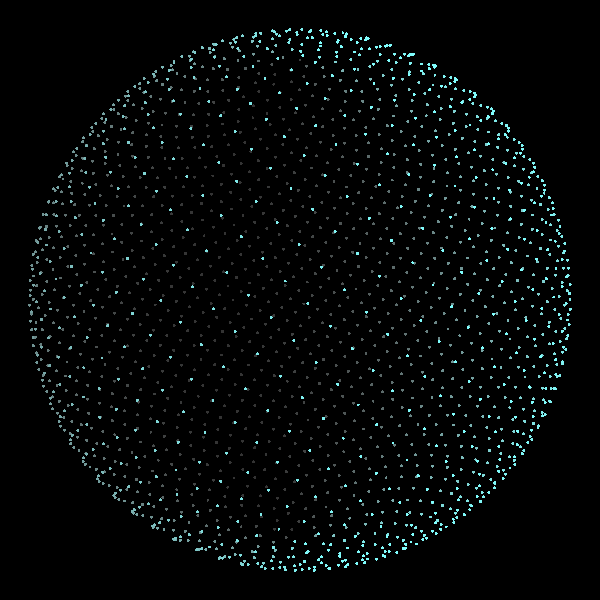# How do I find the correct distance spacing for distributing equidistant points on a sphere of a given diameter?

How do I find the correct distance spacing for distributing equidistant points on a sphere of a given diameter?

I don't need to fill the sphere with equidistant points. I just need less than a hemisphere. Lets say around 100 points packed together at equidistant distances between 0.1 inches and 0.2 inches on a sphere with a diameter of 1.25 inches. The spacing cannot vary more than 0.001".

For some ready-to-use code (with a demo, see below), you can use the Fibonacci algorithm. (Click on the $$$$ sign at the top of that page to see the code.)demo by Jim Bumgardner